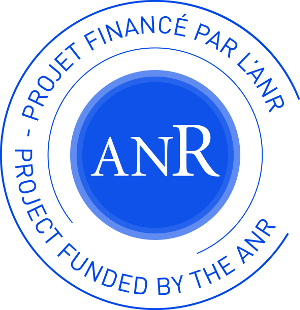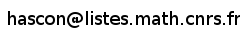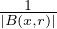Project ANR JCJC HASCON (2019 - 2023)

Harmonic Analysis for Semigroups on Commutative and Non-commutative Lp spaces

Version française

Contact :### 1 Members

• Cédric Arhancet
• Luc Deleaval
• Adrián González-Pérez (postdoctorant year 2019-2020)
• Christoph Kriegler (project coordinator)

### 2 Key words of research

• Harmonic Analysis
• Semigroups of operators
• Non-commutative geometry
• Functional calculus
• Maximal operators
• Non-commutative Lp spaces
• Operator spaces
• Riesz transforms
• Singular integrals
• Geometry of Banach spaces
• Fourier multipliers on groups and Schur multipliers
• R-boundedness

### 3 Presentation of the project

Since the fundamental works of Stein and Cowling, the spectral theory for semigroups has become a wide mathematical field and a lot of mathematicians work in that field today. Much progress has been achieved over the last four decades, many beautiful connections have been proven to be fruitful in solving problems inside and outside harmonic analysis. The aim of our project HASCON is to answer the following questions, which arise in the context of spectral theory, functional calculus, harmonic analysis or abstract partial differential equations:

1. Under which circumstances (e.g. which underlying classical or non-commutative Lp or Banach space) does the generator of a semigroup admit an H or Hormander(-Mihlin) functional calculus? This is a property well-known to be of great importance in theoretical aspects and for many applications. The answer depends on the underlying space X, which can consist of functions over some measure space Ω (often Lp(Ω)), or also be a non-commutative Lp space, i.e. a space of (un)bounded operators affiliated with a von Neumann algebra. Hereby, geometrical properties of the Banach space X usually play an important rôle, and we give a particular emphasis to Bochner spaces X = Lp,Y ). Then the property of Y being a UMD space becomes important, and also its Rademacher-type and -cotype as well as related notions such as p-convexity and q-concavity if Y is moreover a lattice. Our motivation for Bochner spaces comes from their importance in applications to abstract Cauchy problems, where Y takes over the rôle of a spatial variable, whereas the time variable is the parameter t of the semigroup Tt; for square function estimates, where Y = 2 (then the interesting functional calculus question involves a sequence of spectral multipliers (fk)k); and lastly for descriptions of abstract function spaces associated with the generator, such as Sobolev and Triebel-Lizorkin spaces, where Y = q.
2. For which cases is an evolution maximal operator, in the most classical form MTf = supt>0|Ttf| or spatial maximal operator MHLf = supr>0B(x,r)|f(y)|dy bounded? This and the above functional calculus question are closely linked and reinforce each other. Namely, on the one hand, an H calculus with a good angle allows to extend the boundedness of the evolution maximal operator on Lp(Ω) above to a sectorial maximal operator. Then under the presence of integral kernel estimates of Tt, evolution and spatial maximal operators are simultaneously bounded. On the other hand, boundedness of a maximal operator plays sometimes a crucial tool in establishing H calculus and Hormander-Mihlin calculus. Again we pay a particular attention to Bochner-space valued boundedness of MHL,MT. From a general point of view, standard maximal operators are important in several branches of harmonic and real analysis (e.g. singular integrals, multipliers, Littlewood-Paley theory).
3. What kind of operations on non-commutative Lp spaces yield bounded and completely bounded maps? The most prominent examples of such mappings important in harmonic analysis are Schur multipliers, non-commutative Fourier multipliers or operations stemming from second quantization, such as q-Ornstein-Uhlenbeck semigroups. Schur multipliers provide a surprisingly rich class of mappings and they have a longstanding usage in various fields of analysis such as complex function theory, Banach spaces, operator theory, multivariate analysis, theory of absolutely summing operators and functional calculus. Fourier multipliers on non-commutative groups and second quantizations are a rather new field in harmonic analysis. Non-commutative harmonic analysis involves more algebraic and also combinatoric structure.

### 4 Events

There has been a two days meeting of the ANR project Thursday 20 October - Friday 21 October 2022 entitled

Harmonic Analysis of semigroups on commutative and non-commutative Lp spaces.

It took place at Université Gustave Eiffel, Marne-la-Vallée, France. The aim of the conference was to bring together mathematicians working on harmonic analysis techniques which have been proven fruitful in the Lp-theory of operators (functional calculus, operator semigroups, maximal operators, square functions) – and in recent times could be successfully adapted to non-commutative counterparts such as Fourier multipliers on l.c. groups and Schur multipliers. There were four presentations of 60 min and seven of 30 min, and as usual also time for discussions was left. The schedule was the following.

 Thursday 20 October 2022 9h00 – 9h30 Reception of the participants at LAMA = coffee break 1 9h30 –10h30 Wolfgang Arendt 10h45 – 11h45 Mariusz Mirek 12h00 – 14h00 Lunch 14h00 –14h30 Blazej Wróbel 14h30 – 15h00 Oliver Dragičević 15h00 – 15h30 Coffee break 2 15h30 – 16h00 Emiel Lorist 16h00 – 16h30 Nick Lindemulder 16h30 – 17h00 Tomasz Szarek 20h00 Conference dinner Thursday 21 October 2022 09h30 – 10h30 El Maati Ouhabaz 10h30 – 11h00 Coffee break 3 11h00 – 11h30 Cédric Arhancet 11h30 – 12h00 Léonard Cadilhac 12h00 – 14h00 Lunch 14h00 – 15h00 Mark Veraar

The following is the list of participants as of 28/09/2022.

1. Benjamin Arras
2. Sebastian Bechtel
3. Clément Coine
4. Lukas Hagedorn
5. Kamal Khalil
6. Fatima Zahra Lahbriri
7. Christian Le Merdy
8. Éric Ricard
9. Silvia Romanelli
10. Elizabeth Strouse
11. Alexandre Thorel

### 5 Publications and Preprints

1. L. Deléaval and C. Kriegler: Dunkl spectral multipliers with values in UMD lattices, Journal of Functional Analysis, 272(5):2132–2175, 2017. Preprint here and on HAL.
2. L. Deléaval and C. Kriegler: Dimension free bounds for the vector-valued Hardy-Littlewood maximal operator, Rev. Mat. Iberoam., 35(1):101–123, 2019. Preprint on HAL, arxiv.org.
3. L. Deleaval, M. Kemppainen and C. Kriegler: Hörmander functional calculus on UMD lattice valued Lp spaces under generalised Gaussian estimates, Journal d’Analyse Mathématique, 145(1), 177–234, 2021. Preprint here, on HAL and on arXiv.org.
4. C. Arhancet and C. Kriegler: Complementation of the subspace of radial multipliers in the space of Fourier multipliers on n, Archiv der Mathematik, 112(1), 93–100, 2019. Preprint on HAL, arXiv.org.
5. C. Arhancet and C. Kriegler: Projections, multipliers and decomposable maps on noncommutative Lp-spaces, to appear in Mémoires de la Société Mathématique de France, 177 (new series). Preprint on HAL and on arXiv.org.
6. C. Arhancet and C. Kriegler: Riesz transforms, Hodge-Dirac operators and functional calculus for multipliers, Springer Lecture Notes in Mathematics, 2304. Springer, 2022, Cham, xii+278 pp. Preprint on HAL and on arXiv.org.
7. C. Arhancet: Dilations of markovian semigroups of Fourier multipliers on locally compact groups, Preprint on arXiv.org.
8. K. Domelevo, C. Kriegler and S. Petermichl: H calculus for submarkovian semigroups on weighted L2 spaces Mathematische Annalen, 381(3-4), 1137–1195, 2021. Preprint on HAL and arXiv.org.
9. C. Arhancet: Positive contractive projections on noncommutative Lp-spaces, Preprint on arXiv.org.
10. C. Arhancet: Contractively decomposable projections on noncommutative Lp-spaces, Preprint on arXiv.org.
11. C. Arhancet: Dilations of markovian semigroups of measurable Schur multipliers, Preprint on arXiv.org.
12. J. Conde-Alonso, A. González-Pérez and J. Parcet: Noncommutative strong maximals and almost uniform convergence in several directions, Preprint on arXiv.org.
13. L. Deléaval, N. Demni: Generalized Bessel functions of dihedral-type: expression as a series of confluent Horn functions and Laplace-type integral representation, The Ramanujan journal, to appear.
14. S. Ben Saïd., L. Deléaval: Translation operator and maximal function for the (k,1)-generalized Fourier transform, submitted.
15. C. Arhancet: Contractively decomposable projections on noncommutative Lp-spaces, submitted.
16. C. Arhancet: Positive contractive projections on noncommutative Lp-spaces, submitted.
17. C. Arhancet and Yves Raynaud: 2-positive contractive projections on noncommutative Lp-spaces, submitted.
18. C. Arhancet: A characterization of completely bounded normal Jordan *-homomorphisms on von Neumann algebras, submitted.
19. C. Arhancet: Quantum information theory and Fourier multipliers on quantum groups, submitted.
20. L. Deléaval and C. Kriegler: Maximal Hörmander Functional Calculus on Lp spaces and UMD lattices, International Mathematical Research Notices, Preprint on HAL, on arXiv.org and here.

Website updated on 23/02/2023.